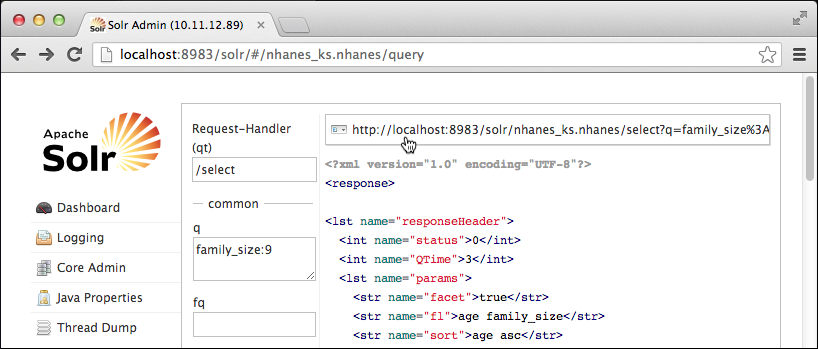# Search the data: Solr HTTP API

Advanced tutorial steps to use the Solr HTTP API to run DSE Search queries.

These advanced tutorial steps use the Solr HTTP API to run search queries. The Solr Admin query form is limited, but useful for learning about Solr, and helps you get started using the Solr HTTP API. The queries in Solr HTTP format appear at the top of the form. After looking at a few URLs, try constructing queries in Solr HTTP format.

## Procedure

To get started using the Solr HTTP API:
1. Scroll to the top of the form, and click the greyed out URL.A page of output, independent of the query form, appears that you can use to examine and change the URL. The URL looks like this:
``````http://localhost:8983/solr/nhanes_ks.nhanes/select?
q=family_size%3A9&sort=age+asc&fl=age+family_size
&wt=xml&indent=true&facet=true&facet.field=age``````
2. In the URL in the address bar, make these changes:

FROM:

``````q=family_size%3A9
&fl=age+family_size
``````

TO:

``````q=age:[20+TO+40]
&fl=age+family_size+num_smokers``````

The modified URL looks like this:

``````http://localhost:8983/solr/nhanes_ks.nhanes/select?
q=age:[20+TO+40]&sort=age+asc&fl=age+family_size+num_smokers
&wt=xml&indent=true&facet=true&facet.field=age``````

In the Solr Admin query form, you can use spaces in the range [20 TO 40], but in the URL, you need to use URL encoding for spaces and special characters. For example, use + or %20 instead of a space, [20+TO+40].

3. Use the modified URL to execute the query. Move to the end of the URL, and press ENTER.

The number of hits increases from 186 to 7759. Results show the number of smokers and family size of families whose members are 20-40 years old. Facets show how many people fell into the various age groups.

``````. . .
</doc>
</result>
<lst name="facet_counts">
<lst name="facet_queries"/>
<lst name="facet_fields">
<lst name="age">
<int name="23">423</int>
<int name="24">407</int>
<int name="31">403</int>
<int name="30">388</int>
<int name="40">382</int>
<int name="28">381</int>
<int name="27">378</int>
<int name="21">377</int>
<int name="33">377</int>
<int name="22">369</int>
<int name="29">367</int>
<int name="20">365</int>
<int name="32">363</int>
<int name="34">361</int>
<int name="36">361</int>
<int name="25">358</int>
<int name="26">358</int>
<int name="35">358</int>
<int name="38">353</int>
<int name="37">339</int>
<int name="39">291</int>
<int name="17">0</int>
. . .``````